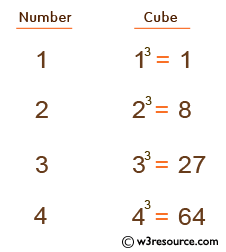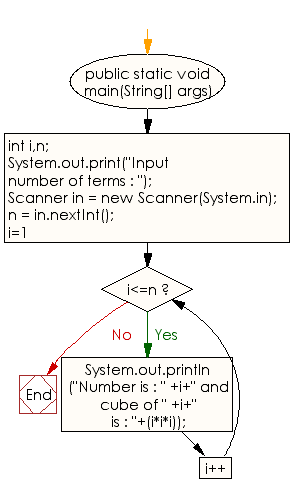﻿ Java exercises: Display the cube of the number upto given an integer - w3resource# Java Conditional Statement Exercises: Display the cube of the number upto given an integer

## Java Conditional Statement: Exercise-13 with Solution

Write a program in Java to display the cube of the number upto given an integer.

Test Data
Input number of terms : 4

Pictorial Presentation:Sample Solution:

Java Code:

``````import java.util.Scanner;
public class Exercise13 {

public static void main(String[] args)

{
int i,n;

System.out.print("Input number of terms : ");
Scanner in = new Scanner(System.in);
n = in.nextInt();

for(i=1;i<=n;i++)
{
System.out.println("Number is : " +i+" and cube of " +i+" is : "+(i*i*i));
}
}
}
```
```

Sample Output:

```Input number of terms : 4
Number is : 1 and cube of 1 is : 1
Number is : 2 and cube of 2 is : 8
Number is : 3 and cube of 3 is : 27
Number is : 4 and cube of 4 is : 64
```

Flowchart:Java Code Editor: